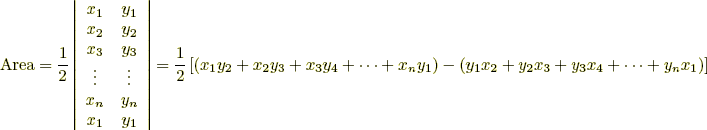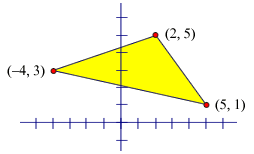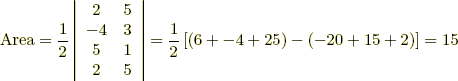index: click on a letter A B C D E F G H I J K L M N O P Q R S T U V W X Y Z A to Z index index: subject areas numbers & symbols sets, logic, proofs geometry algebra trigonometry advanced algebra & pre-calculus calculus advanced topics probability & statistics real world applications multimedia entrieswww.mathwords.com about mathwords website feedback

Area of a Convex Polygon

The coordinates (x1, y1), (x2, y2), (x3, y3), . . . , (xn, yn) of a convex polygon are arranged in the "determinant" below. The coordinates must be taken in counterclockwise order around the polygon, beginning and ending at the same point.Example: Find the area of this polygon: